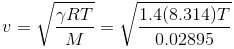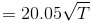﻿ All the formulas related to Sound Waves

# List of formulas related to Sound Waves

### Sound Speed

V (m/s): sound speed in environment with temperature t (celsius)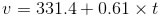### Sound Intensity

E: sound energy (J), P: sound power (W), A: the surface area of a sphere (m2), t: time (s)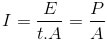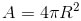### Sound Intensity Level

I0: the standard reference sound intensity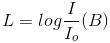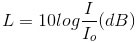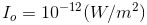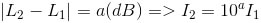### The Doppler Effect

Vo: the relative speed of the observer; vs: the relative speed of the source; v: the sound speed in this medium; fs, fo: sound frequency of the source and observer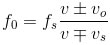### Sound Speed In Gases

V: speed of sound (m/s), R: universal gas constant, T: absolute temperature (K), M:molecular mass of gas (kg/mol), γ: adiabatic constant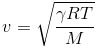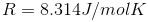Example in air